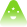Getting Started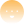Learn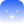Use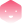Build
learn

# Interest Rate Methodology

Contributors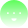Tinlake uses an interest rate mechanism that is typically implemented as compounding per second. The implementation can be found in github.com/centrifuge/tinlake-math.

Below we show abstract examples of how this is calculated:

Variable
Description
$P$
Principal
$D$
Debt
$rate$
interest rate per second
$n$
the number of times the interest is compounded, compounding is once per second, so "n" is seconds past since last calculation
$mathtt{R}$
Nominal interest rate (5% would be 0.05)
$mathtt{A}$
Annual Percentage Rate (APR)
$mathtt{y}$
constant, seconds in a year: $3600 cdot 24 cdot 365 = 31536000$

The basic formula is:

$D = P cdot rate^{n}$

Debt equals to Principal (or the previous debt amount) times rate to the power of seconds since last calculation.

## Example: Interest rate compounding per second

$P = 100 ewline r = 0.05 ewline n = 3600 cdot 24 cdot 365 = 31536000 ewline$

Using the formula above, the Debt $D$ after half a year $(n = mathtt{y} / 2 = 15768000)$ would result in $D = 102.5315$.

After one year ($n = mathtt{y}$) the $D$ would be $105.1271$.

Thus a 5.00% interest rate compounded every second is equivalent to an annually compounded rate of 5.127%.

The annual percentage rate $mathtt{A}$ could also be calculated directly from the percentage rate $mathtt{R}$ (using $n = mathtt{y} = 31536000$):

$mathtt{A} = (1 + rac{mathtt{R}}{mathtt{y}})^mathtt{y} = 1.05127$

### Rate per Second

To calculate the Debt, we initialize an interest rate in Tinlake with a variable called ratePerSecond or $rate$. The ratePerSecond represents the interest accrued per second in Tinlake.

$rate = 1 + rac{mathtt{R}}{mathtt{y}}$

### Calculate Debt

$D = P * rate^t$

The debt can be calculated by multipling the principial $P$ with $rate$ to the power of $n$. The variable $n$ represents the time passed in seconds since the loan has been borrowed.

Continuing the example from above for annual interest:

$rate = 1 + rac{0.05}{31536000} = 1.0000000015854900 ewline D = 100 cdot 1.0000000015854900^{31536000} = 105.1271$

## Using an annual percentage rate (APR) in Tinlake

The Tinlake User Interface uses an annual percentage rate (APR) as input. Tinlake transforms this annually compounded rate $mathtt{A}$ into the equivalent rate used for compounding per seconds $rate$. This is achieved by solving the equation:

$mathtt{A} = (1 + rac{rate}{mathtt{y}})^mathtt{y}$

for $r$:

$r = mathtt{y} cdot (mathtt{A}^{ rac{1}{mathtt{y}}}-1)$

Using the calculated $r$ compounding every second leads to the same amount of debt like using $mathtt{A}$ compounding annually over the course of a year. Thus, the calculated rate can be used to achive an interest per year (APR) behaviour with the compounding per second implementation in Tinlake.

Continuing the example from above with an 5.00% annual interest rate (APR):

## Decimal Precision

We use fixed precision decimals for any monetary amounts. Interest Rates are typically of type ray with 27 digits precision and amounts are of type wad which has 18 digits precision.

This is usually explicitally mentioned in throught the codebase.